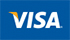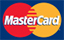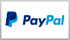Produce

View as Grid List

Items 1-90 of 128

Page
per page
Set Descending Direction
1. apples gold
J\$209.48
2. apples red
J\$170.61
3. Apples Red Import Perry Kg Tray
J\$276.01
J\$991.22
J\$811.00
6. Baby Carrots per kg
J\$587.72
7. bean sprout
J\$1,070.56
8. Beet Root per kg
J\$169.93
9. Black plums per kg
J\$1,628.15
10. Black Seedless Grapes per kg
J\$2,416.94
11. blueberries 170gm
J\$1,642.34
12. Bosc Pear per lb
J\$1,132.02
13. Broccoli Crowns per kg
J\$2,326.53
14. broccoli local
J\$350.75
15. cabbage (per kg)
J\$396.51
16. callaloo (per kg)
J\$212.25
17. callaloo shredded
J\$240.30
18. Canteloupe Local per kg
J\$346.05
19. carrot local (per kg)
J\$370.08
20. Cauliflower Local (per kg)
J\$509.80
21. Celery Naked (per kg)
J\$290.09
22. Celery per kg
J\$481.28
23. Cho Cho Local per kg
J\$377.59
24. chocolate balls
J\$119.56
25. cinnamon leaves
J\$102.99
26. clean x angle brooms dustpan (per kg)
J\$246.54
27. coco (per kg)
J\$475.47
28. corn local
J\$433.93
29. D'Anjou Pear per lb
J\$1,828.64
30. dasheen (per kg)
J\$428.21
31. dd herb fresh spinach
J\$463.43
32. dried peanuts (per kg)
J\$1,330.96
33. Dry Coconut per kg
J\$206.93
34. Eggplant Local per kg
J\$101.15
35. escallion (per kg)
J\$203.92
36. french lime
J\$595.04
37. fresh kale
J\$408.98
38. fruit dish (per kg)
J\$1,187.76
39. Fuji Apple per kg
J\$1,229.40
40. Gala Apples perr kgg
J\$1,398.45
41. Gala Apples KG
J\$1,158.69
42. garlic (per kg)
J\$105.91
43. geddy ss dried gungo peas 400gm
J\$137.68
44. goya black eye peas 397gm
J\$355.48
45. goya pigeon peas 397gm
J\$471.33
46. goya yellow split peas 397gm
J\$201.76
47. Granny Smith Apples per kg
J\$1,087.46
48. Grapefruit
J\$308.96
49. green banana (per kg)
J\$293.13
50. Green plantain per kg
J\$604.87
51. Green Seedless Grapes per kg
J\$2,250.67
52. Hominy Corn Bulk per kg
J\$105.97
53. honey dew melon local (per kg)
J\$449.52
54. Hot Pepper Local per kg
J\$186.98
55. Irish Potato
J\$603.81
56. Irish Potato (per kg)
J\$491.65
57. Irish Potato per Kggg
J\$608.90
58. Irish Potatoes per kg
J\$774.86
59. JD Spice Whole Nutmeg 45g
J\$274.63
60. Jelly Coconut
J\$205.97
61. June Plum per kg
J\$343.29
62. kiwi (per kg)
J\$2,146.60
63. lemon
J\$600.74
64. Lettuce Local Per Kg
J\$348.32
65. Lettuce per kg
J\$257.70
66. lime
J\$343.29
67. local round red peas (per kg)
J\$221.89
68. local yellow squash (per kg)
J\$428.21
69. local zucchini (per kg)
J\$523.67
70. melon (per kg)
J\$391.25
71. Mustard Spinach per kg
J\$377.63
72. Naseberry per kg
J\$240.30
73. Okra (per kg)
J\$292.15
74. Onion perrkgg
J\$439.00
75. onion red (per kg)
J\$769.29
76. Onions per kg
J\$486.18
77. Onions per kggg
J\$295.01
78. Onions perrkggg
J\$583.15
79. Oranges Local Per Dozen
J\$514.93
80. Pak Choi (per kg)
J\$193.74
81. Pak Choy per kg
J\$441.02
82. peanut grain (per kg)
J\$1,901.90
83. pears bosc 80 110 19 1kg (per kg)
J\$1,579.97
84. pepper scotch bonnet
J\$102.99
85. Peppers Hot Jalapeno per kg
J\$880.22
86. phf bulgar
J\$204.08
87. picker s choice green pepper
J\$350.17
88. picker s choice red pepper
J\$350.17
89. picker s choice yellow pepper
J\$350.17
90. pimento
J\$92.68
View as Grid List

Items 1-90 of 128

Page
per page
Set Descending Direction

Payment Option

•••